ISEE Middle Level Math : Numbers and Operations

Example Questions

Example Question #9 : How To Find The Part From The Whole

Angelina goes to the grocery store. She only has $13.50 to spend. How many gallons of milk can she buy if each costs$4.50?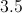Explanation:

Divide: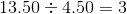Example Question #10 : How To Find The Part From The Whole

Diana sold seven of her collectable dolls and made $350.00. How much was each doll sold for? Possible Answers: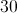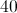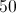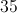Correct answer:Explanation: Divide: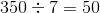Answer: Each doll was sold for$50.00.

Example Question #11 : Whole And Part

Ninety-six people must form eight equal groups. How many people will be in each group?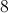Explanation:

Divide: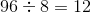Answer: There will be 12 people in each group.

Example Question #51 : Numbers And Operations

Three friends are in the desert and must share a 15 ounce bottle of water. How many ounces of water will each friend drink, assuming they split the bottle evenly?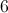Explanation:

Divide: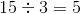Answer: Each friend will drink 5 ounces of water.

Example Question #51 : Numbers And Operations

Pamela takes her children to the fair. How many $3.00 tickets can she buy if she only has$21.00?Explanation:

Divide: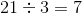Example Question #11 : How To Find The Part From The Whole

In March, there were 10 sunny days, 10 cloudy days, and 11 rainy days. How many days did it rain in March?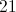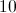Explanation:

Identify the number of rainy days in March:days

Example Question #52 : Numbers And Operations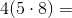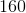Explanation:

Solve inside the parentheses: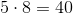Then, solve: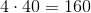Example Question #16 : Whole And Part

Sal is going to the fair with his family. He has $18.00 to buy tickets. How many tickets can he buy if each one costs$2.25?Explanation:

Divide the total amount of money he has by the cost of one ticket: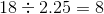Example Question #53 : Numbers And Operations

Julie gets paid $304 a week for dogsitting. If she wants to buy a new washing machine that costs$1400, how many weeks does Julie need to work to save up the required amount? Round to the nearest whole week.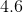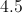Explanation:

Divide the cost of the washing machine by the amount of money she makes each week: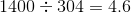Answer: Julie needs to save for 5 weeks.

Example Question #21 : How To Find The Part From The Whole

In order to travel from New York to Florida with his family, Eric needs to purchase 3 tickets, costing $52.00,$64.79 and $44.50. If he has$213.00 in his wallet, how much money will Eric have left over after buying the tickets?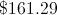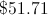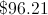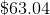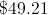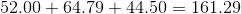Then subtract that amount from $213: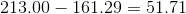Therefore Eric will have$51.71 left over.